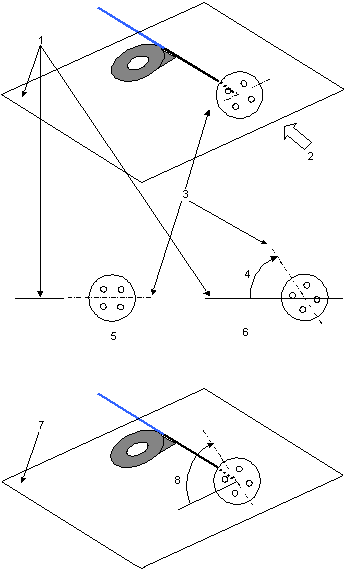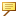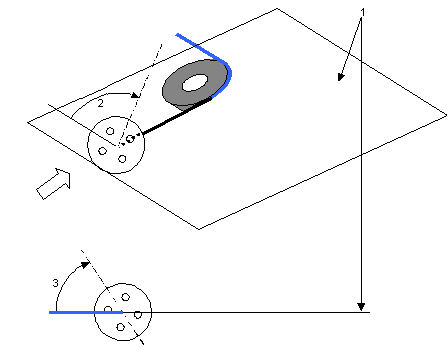Flanges are welded onto a pipe before bending. The pipe must be rotated through an angle before bending so that after bending, the flange bolt holes are at the correct position.
The installation position of a pipe with a flange welded at one of its ends is shown in the following figure. The z-axis and the black segment form the flange base plane. The black and blue segments of the pipe form the bending plane. The remainder of the angle between the flange base plane and the bending plane divided by the angle between the flange holes is the flange angle.1. Flange angle
2. Flange base plane
3. Bending plane
If the black segment is along the z-axis, then the flange base plane is formed by the y-axis and the black segment.
The next figure shows a flange welded onto the straight pipe.1. Flange baseline
After welding the flange onto the pipe, you must load the pipe on the bending machine. When you load the pipe on the bending machine, first align the flange base plane with the bending plane, then rotate the flange with the given flange angle. Standing in front of the bending machine, looking into the pipe when the rotation is in the clockwise direction, the value of the flange angle is negative. When the rotation is in the counterclockwise direction, the value of the flange angle is positive.1. Bending plane
2. Looking into the bending machine
3. Flange base plane
4. Flange angle
5. Before flange angle rotation
6. After flange angle rotation
7. Bending plane
8. Flange angleThe flange base plane aligns with the z-axis when you put the pipe at its installation position.
The pipe is ready for the first bending after the flange angle rotation. The first bending is shown in the next figure.1. Bending plane
2. Flange angle
3. Flange angle